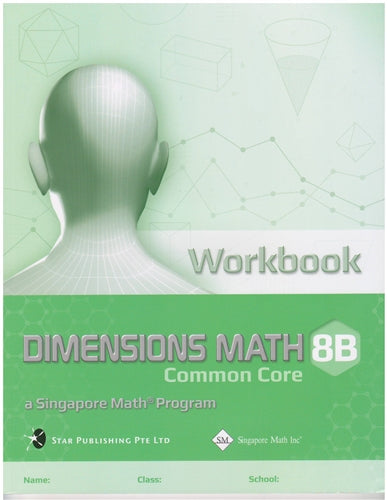# Singapore Math Dimensions Math Workbook 8B

Workbooks are the essential supplements to textbooks as they give students the opportunity to practice applying new concepts. Workbook exercises polish analytical skills and help students develop a stronger foundation in math through repetition and variation.

Features & Components:

• Basic Practice: Simple questions that drill comprehension of concepts.
• Further Practice: More difficult questions that involve direct application.
• Challenging Practice: Questions that require synthesis.
• Enrichment: Questions that demand higher order thinking, analysis, and reasoning.

The answer key at the back of the book provides answers only. Worked Solutions (sold separately) contain fully worked solutions.

Note: Two workbooks (A and B) correspond to the two halves of the school year. Soft cover.

Dimensions Math® Workbook 8B

Chapter 8: Graphs of Linear and Quadratic Functions

8.1 Linear Function

A. Idea of Functions
B. Linear Functions
C. Rate of Change

A. Graph of y = ax2 + bx + c, where a > 0
B. Graph of y = ax2 + bx + c, where a < 0

In a Nutshell
Review Exercise 8

Chapter 9: Graphs in Practical Situations

9.1 Tables, Charts and Graphs
9.2 Distance-Time Graphs

In a Nutshell
Review Exercise 9

Chapter 10: Pythagorean Theorem

10.1 Pythagorean Theorem
10.2 The Converse of Pythagorean Theorem

A. Definition
B. Determination of Right-Angled Triangles

10.3 Applications of Pythagoras Theorem

In a Nutshell
Review Exercise 10

Chapter 11: Coordinate Geometry

11.1 Distance Between Two Points
11.2 Slope of a Straight Line
11.3 Equation of a Straight Line

In a Nutshell
Review Exercise 11

Chapter 12: Mensuration of Pyramids, Cylinders, Cones and Spheres

12.1 Pyramids

A. Introducing Pyramids
B. Nets and Surface Areas of Pyramids
C. Volumes of Pyramids

12.2 Cylinders

A. Volume and Surface Area of a Cylinder

12.3 Cones

A. Introducing Cones
B. Net and Surface Area of a Cone
C. Volume of a Cone

12.4 Spheres

A. Introducing Spheres
B. Volume of a Sphere
C. Surface Area of a Sphere

In a Nutshell
Review Exercise 12

Chapter 13: Data Analysis

13.1 Organizing Data in Frequency Tables
13.2 Bar Graphs and Histograms

A. Bar Graphs
B. Histograms

13.3 Line Graphs and Scatter Plots

A. Line Graphs
B. Scatter Plots

In a Nutshell
Review Exercise 13

14.1 Solving Quadratic Equations by Factorization
14.2 Completing the Square Method
14.4 Graphical Method

In a Nutshell Review Exercise 14